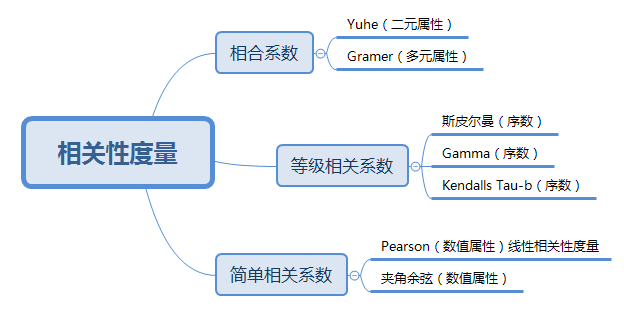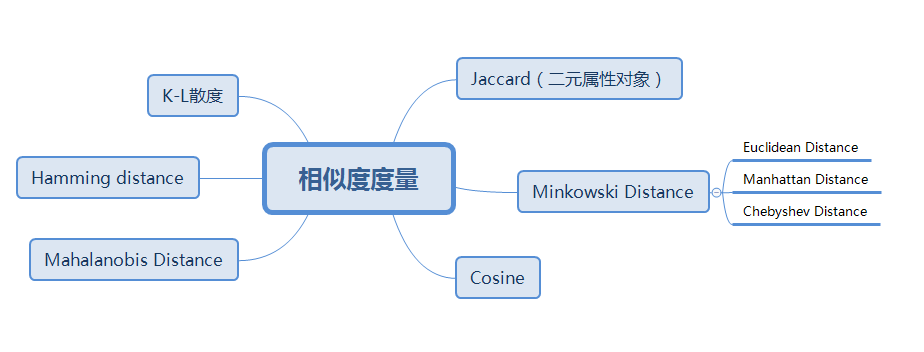# 1. 简介

## 1.1 动作识别分类

• 基于深度信息 （相关论文）
• 3d action recognition from novel viewpoints (2016)
• Global context-aware attention lstm networks for 3d action recognition (2017)
• Super normal vector for activity recognition using depth sequences. (2012)
• Joint angles similarities and hog2 for action recognition (2013)
• Unsupervised learning of view-invariant action representations (2018)
• Depth pooling based large-scale 3-d action recognition with convolutional neural networks （2018）
• Action recognition for depth video using multi-view dynamic images （2019）
• 基于骨骼信息（相关论文）
• Skeleton-based human action recognition with global context-aware attention lstm networks (2017)
• An attention enhanced graph convolutional lstm network for skeleton-based action recognition (2019)
• Actional-structural graph convolutional networks for skeleton-based action recognition (2019)
• View adaptive neural networks for high performance skeleton-based human action recognition (2019)
• Twostream adaptive graph convolutional networks for skeletonbased action recognition (2019)
• Skeleton-based action recognition with directed graph neural
networks (2019)

## 1.2 人体姿态相似性度量

• 进行姿态相似性分析、动作识别、动作分类、关键帧提取等工作大多数都是首先基于关节点的位置进行构造人体姿态特征，然后再基于构造的姿态特征进行动作的识别或者理解等下一阶段工作。
• 人体姿态相似性度量的主要工作包括两个阶段：
• 人体姿态特征构造：表征人体姿态，评估不同人体姿态之间的差异
• 人体姿态度量学习
• 人体姿态特征构造可基于：
• 关节点
• 部位聚类特征点
• 免去了训练回归模型从聚类特征点回归到关节点的步骤，减少了计算量，提高了效率

## 1.3 向量内积（点乘$\odot$）结果为标量

• 定义
要求：a与b的列数相同，记$a=[a_1, a_2, ..., a_n]^T \quad b=[b_1, b_2, ..., b_n]^T$
$[a, b] = a \odot b = a^Tb = \sum_{i=1}^n a_ib_i$
• $\theta$为向量a与b的夹角，则有：
$a \odot b = \vert a \vert \vert b \vert cos \theta$
• $|a|$：表法n维向量a的长度
$|a| = \sqrt {a_1^2 + a_2^2 + ... + a_n^2}$
• 向量a与b正交的充要条件：$a \odot b = 0$
• 几何意义
• 表征或计算两个向量之间的夹角
$\theta = arccos (\frac {a \odot b}{|a| \, |b|})$
• $a \odot b > 0$：方向基本相同，夹角在0°到90°之间
• $a \odot b = 0$：正交，相互垂直
• $a \odot b < 0$：方向基本相反，夹角在90°到180°之间
• b向量在a向量方向上的投影

## 1.4 向量外积（叉乘$\otimes$）： 结果为向量

• 定义：
$a=(x_1, y_1, z_1), b=(x_2, y_2, z_2)$
$a \otimes b = \begin{bmatrix} i & j & k \\ x_1 & y_1 & z_1 \\ x_2 & y_2 & z_2 \end{bmatrix} \\ = (y_1 z_2 - y_2 z_1) i - (x_1 z_2 - x_2 z_1) j + (x_1 y_2 - x_2 y_1) k$
• $i=(1, 0, 0) \quad j=(0,1,0) \quad k=(0, 0, 1)$
$a \otimes b = (y_1 z_2 - y_2 z_1, - (x_1 z_2 - x_2 z_1), x_1 y_2 - x_2 y_1)$
• 性质
$a \otimes b = - b \otimes a$
• 几何意义
• 三维空间
• 向量a和向量b的叉乘结果是一个向量
• 此向量是法向量，即该向量垂直于a和b向量构成的平面
• 二维空间
• $|a \otimes b|$等于由向量a和向量b构成的平行四边形的面积。

# 2. 基于部位聚类特征的人体姿态相似性度量

## 2.1 基于部位聚类点的姿态特征构造

• 姿态特征组成元素
• 姿态特征均由点、线、面等构成的距离、角度、方向等几何信息构成
• 点(Point)
• 点的信息主要是指部位内的聚类特征点，每个点P 用对应的三维空间坐标$(p_x, p_y, p_z)$ 来表示
• 当每个部位内聚类的点为3 个时，一共5 个部位，这样的聚类特征点算上中心点，一共16 个
• 当每个部位内聚类的点为4 个时，这样的聚类特征点一共21 个
• 线(Line)
• 线主要是指不同的聚类特征点之间的连线
• 相邻特征点的连线
• 中心点到肢体端点的连线
• 肢体端点间的边线
• 面(Surface)
• 由各个身体部位上的特征点拟合的平面
• 主要有4个平面的信息，分别是人体四肢构成的平面信息
• 特征表示：
• 特征点的坐标：表示特征点在三维空间中的坐标信息
$f_{P\_c}(P) = (p_x, p_y, p_z)$
• 特征点间的距离：表示两个特征点之间的欧氏距离
$f_{PP\_d} (P_1, P_2) = \Vert \overrightarrow{P_1P_2} \Vert$
• 特征点间的方向向量：表示特征点$P_1$$P_2$ 方向的单位向量
$f_{PP\_v} (P_1, P_2) = unit( \overrightarrow{P_1P_2})$
• 特征点到线的距离：表示特征点P 到特征点$P_1$ 与特征点$P_2$ 组成的直线的距离
$f_{PL\_d}(P, L_{P_1 \to P_2}) = 2S_{\bigtriangleup PP_1P_2} /f_{PP\_d}(P_1, P_2)$
• $S_{\bigtriangleup PP_1P_2}$ ：表示$P ， P_1 ， P_2$ 三个点围成的三角形的面积
• 直线与直线的夹角：表示聚类特征点之间构成的直线$L_{P_1 \to P_2}$$L_{P_3 \to P_4}$之间的夹角，夹角的取值范围是$[0, \pi]$
$f_{LL\_\alpha} (L_{P_1 \to P_2}, L_{P_3 \to P_4} ) = arccos(f_{PP\_v} (P_1, P_2) \odot f_{PP\_v} (P_3, P_4))$
• $\odot$：表示两个向量之间点乘运算
• 特征点到平面的距离：特征点P 到由特征点$P_1 ，P_2 ，P_3$ 组成的平面的距离
$f_{PM\_d}(P, M_{P_1 \to P_2 \to P_3}) = \\ f_{PP\_v}(P_1, P) \odot unit( f_{PP\_v}(P_1, P_2) \otimes f_{PP\_v}(P_1, P_3))$
• $\otimes$：表示两个向量之间的叉乘运算
• 直线与平面的夹角：表示特征点$P_1$$P_2$之间的连线与$P_3 ， P_4 ， P_5$ 三个特征点拟合的平面之间的夹角，其范围为$[0, \pi]$
$f_{LM\_\alpha} (L_{P_1 \to P_2}, M_{P_3 \to P_4 \to P_5})=\\ arccos(f_{PP\_v(P_1, P_2)} \odot unit (f_{PP\_v(P_3, P_4)} \otimes f_{PP\_v(P_3, P_5)}))$
• $f_{PP\_v(P_3, P_4)} \otimes f_{PP\_v(P_3, P_5)}$：表示$P_1, P_2, P_3$三个特征点拟合的平面的法向量
• 平面间的夹角：表示的是$P_1, P_2, P_3$ 三个特征点拟合的平面与$P_4, P_5, P_6$三个特征点拟合的平面之间的夹角
$f_{MM\_\alpha} (M_{P_1 \to P_2 \to P_3}, M_{P_4 \to P_5 \to P_6}) = \\ arccos(unit (f_{PP\_v(P_1, P_2)} \otimes f_{PP\_v(P_1, P_3)}) \odot unit (f_{PP\_v(P_4, P_5)} \otimes f_{PP\_v(P_4, P_6)}))$

## 2.2 相似度、相关性度量指标

• 相关性：是数据属性相关性的度量方法
• 相似度：是数据对象相似性度量的方法
• 数据对象：由多个数据属性描述
• 数据属性的相关性：由相关系数来描述
• 数据对象的相似度：由某种距离度量
• 许多数据分析算法会涉及相似性度量相关性度量，如聚类、KNN等

### 2.2.1 相关性度量

• 相关性用相关系数来度量
• 相关系数绝对值越大表是相关性越大，相关系数取值在[-1, 1]之间，0表示不相关#### 2.2.1.1 皮尔森相关系数 (Pearson Correlation Coefficient)

• 值越大越相似
• 计算公式：
设向量X和Y分别为：$X = (x_1, x_2, ..., x_n)^T, Y=(y_1, y_2, ..., y_n)^T$
$f_{PCC}(X,Y) = \frac{\sum_{i=1}^n (x_i - \bar X) (y_i - \bar Y)}{\sqrt {\sum_{i=1}^n (x_i - \bar X)^2} \sqrt {\sum_{i=1}^n (y_i - \bar Y)^2}}$

#### 2.2.1.2 余弦相似度 (Cosine Similarity)

• 值越大越相似
• 计算公式：
设向量X和Y分别为：$X = (x_1, x_2, ..., x_n)^T, Y=(y_1, y_2, ..., y_n)^T$
$f_CS(X,Y) = \frac {X \cdot Y}{|X||Y|}$

### 2.2.2 相似度（性）度量

• 相似度：用距离来度量
• 相似度通常是非负的，取值在[0, 1]之间
• 距离越大，相似性越小，在应用过程中要注意计算的是相似度还是距离2) 考虑了模式的分布
3)尺度不变性
1) 马氏距离的计算是不稳定的，不稳定的来源是协方差矩阵，这也是马氏距离与欧式距离的最大差异之处
2)夸大了变化微小的变量的作用
3)在计算马氏距离过程中，要求总体样本数大于样本的维数

#### 2.2.2.1 欧氏距离 (Euclidean Distance)

• 值越小越相似
• 计算公式：
设向量X和Y分别为：$X = (x_1, x_2, ..., x_n)^T, Y=(y_1, y_2, ..., y_n)^T$
$f_{ED} (X,Y)= \sqrt {\sum_{i=1}^n (x_i - y_i)^2)}$
• 计算标准化欧氏距离
• 先将各个维度的数据进行标准化：标准化后的值 = ( 标准化前的值 － 分量的均值 ) /分量的标准差
• 然后计算欧式距离
• 缺点
• 量纲需一致
• 每个坐标对欧氏距离的贡献是同等的。当坐标表示测量值时，它们往往带有大小不等的随机波动，在这种情况下，合理的方法是对坐标加权，使变化较大的坐标比变化较小的坐标有较小的权系数，这就产生了各种距离。当各个分量为不同性质的量时，“距离”的大小与指标的单位有关。它将样品的不同属性（即各指标或各变量）之间的差别等同看待，这一点有时不能满足实际要求。没有考虑到总体变异对距离远近的影响。
• 特点：欧式距离就好比一个参照值，它表征的是当所有类别等概率出现的情况下，类别之间的距离

#### 2.2.2.2 曼哈顿距离 (Manhattan Distance)

• 值越小越相似
• 计算公式：
设向量X和Y分别为：$X = (x_1, x_2, ..., x_n)^T, Y=(y_1, y_2, ..., y_n)^T$
$f_{ManD} (X,Y)= \sum_{i=1}^n \vert x_i - y_i \vert$

#### 2.2.2.3 闵可夫斯基距离(Minkowsk Distance)

• 值越小越相似
• 计算公式：
设向量X和Y分别为：$X = (x_1, x_2, ..., x_n)^T, Y=(y_1, y_2, ..., y_n)^T$
$f_{MinD} (X, Y)= (\sum_{i+1}^n \vert x_i - y_i \vert^p)^{1/p}$
• 当p=1：明可夫斯基距离变成曼哈顿距离
• 当p=2：明可夫斯基距离变成欧几里得距离
• 当p=∞：明可夫斯基距离变成切比雪夫距离
• 缺点:
• 数据量纲不同，无法直接进行距离计算，需要先对数据进行归一化
• 没有考虑各个分量的分布（期望，方差等)

#### 2.2.2.4 马氏距离 (Mahalanobis Distance)

• 值越小越相似
• 计算公式：
设向量X和Y分别为：$X = (x_1, x_2, ..., x_n)^T, Y=(y_1, y_2, ..., y_n)^T$
$f_{MahD}(X, Y) = \sqrt {(X-Y)^T \Sigma^{-1}(X-Y)}$
• $\Sigma$：为X和Y的协方差矩阵，若协方差矩阵是单位矩阵则变为欧式距离
• 协方差矩阵的性质
• 实对称矩阵（Symmetric Matrix）
• 所有特征值都为实数
• 不同特征值对应的特征向量互相正交
• $\Sigma = \Sigma^T$
• $\Sigma = U \land U^T$
• $U$：为正交矩阵($U^{-1}=U^T$)
• $\land$：为对角矩阵，对角线上的值为$\Sigma$的特征值，按从大到小的顺序排列
• 优点
• 与量纲无关、排除变量之间的相关性的干扰
• 修正了欧式距离中各个维度尺度不一致且相关的问题
• 当类别先验概率不相等时，马氏距离中引入的协方差参数（表征的是点的稀密程度）来平衡两个类别的概率
• 问题
• 协方差矩阵必须满秩
• 里面有求逆矩阵的过程，不满秩不行，要求数据要有原维度个特征值，如果没有可以考虑先进行PCA，这种情况下PCA不会损失信息
• 不能处理非线性流形(manifold)上的问题
• 只对线性空间有效，如果要处理流形，只能在局部定义，可以用来建立KNN图
• 相关基础
• 标准差（一维数据）：数据集中各个点到均值点距离的平均值
$\sigma_x$
• 方差（一维数据）：方差是标准差的平方，反应的是数据的离散程度
$\sigma_x^2 = \frac{1}{n-1} \sum_{i=1}^n (x_i - \bar x)^2$
• $n$：表示样本量
• $\bar x$：表示观测样本的均值
• 协方差（多维数据）反应两个随机变量的相似程度
• 当存在多维数据时，我们通常需要知道每个维数的变量中间是否存在关联
• 协方差就是衡量多维数据集中，变量之间相关性的统计量
• 比如：一个人的身高与他的体重的关系，这就需要用协方差来衡量
• 如果两个变量之间的协方差为正值，则这两个变量之间存在正相关，若为负值，则为负相关
• 协方差矩阵（多维数据）：当变量超过两个，就用协方差矩阵来衡量各个变量之间的相关性
• 计算协方差矩阵（多维数据, 方阵，维数等于样本的维数）
• 设有n个样本：$Z_1, Z_2, ..., Z_n$,
• 每个样本有m维数据：$Z_i = (z_{i1}, z_{i2}, ..., z_{im})$
• 则有m个列向量：$X_i = (z_{1,i}, z_{2,i}, ... , z_{n,i})$
• $\mu_i$是第$i$列的期望值：$\mu_i = E(X_i)$
• 协方差矩阵的第$i行j列$为：（计算维度之间的相关性）
$\sum_{i,j} = cov(X_i, X_j) = E[(X_i - \mu_i)^T(X_j - \mu_j)])$
• 即：协方差($i,j$)=$(第i列所有元素 - 第i列均值)^T * (第j列所有元素 - 第j列均值)/（样本数-1）$
• 计算协方差矩阵实例
• 样本
$x_1 = (1, 2,3 ,4)^T \quad x_2=(3, 4, 1, 2)^T \quad x_3=(2, 3, 1, 4)^T$
• 生成如下矩阵
$\begin{bmatrix} 1 & 2 & 3 & 4 \\ 3 & 4 & 1 & 2 \\ 2 & 3 & 1 & 4 \end{bmatrix}$
• 计算各列均值
$u_1=(1+3+2)/3 = 2$
$u_2=(2+4+3)/3 = 3$
$u_3=(3+1+1)/3 = 1.67$
$u_4=(4+2+4)/3 = 3.33$
• 计算协方差矩阵元素：
$\Sigma_{2,3} = [(2, 4, 3)^T - (3, 3, 3)^T]^T \cdot [(3, 1, 1)^T - (1.67, 1.67, 1.67)^T]/(3-1) \\ =(-1, 1, 0)(1.33, -0.67. -0.67)^T/2 = -1$
• 生成的协方差矩阵
$\begin{bmatrix} 1.0 & 1.0 & -1.0 & -1.0 \\ 1.0 & 1.0 & -1.0 & -1.0 \\ -1.0 & -1.0 & 1.3333 & 0.6667 \\ -1.0 & -1.0 & 0.6667 & 1.3333 \\ \end{bmatrix}$

#### 2.2.2.5 海明距离 （Hamming Distance）

• Hamming Distance可以用来度量两个串（通常是二进制串）的距离，其定义为这两个二进制串对应的位有几个不一样，那么海明距离就是几，值越小越相似
• 例如x=1000，y=1111，那么x和y的海明距离就是3
• 信息编码时为了增强容错性，应使得编码间的最小海明距离尽可能大

#### 2.2.2.6 杰卡德相似系数 ( Jaccard Coefficient)

• 用途：度量两个集合的相似度
• 值越大越相似
• 设有两个集合$S_1$$S_2$，它们之间的Jaccard Coefficient定义为：
$f_{JC}(S_1, S_2) = \frac{|S_1 \cap S_2|}{|S_1 \cup S_2 |}$
• 如：$S_1 = {A, B, C, D}, S_2= {B, D, E}$，则$f_{JC}(S_1, S_2) = \frac {2}{5}$

### 2.2.2.7 K-L散度(Kullback-Leibler Divergence)

• 用途： KL散度用来度量两个分布之间的相似度
• 值越小，两个分布就越相似
• 分布P和分布Q的KL散度定义为
$f_{KL}(P||Q) = \sum_{i=1}^n P(x_i)log\frac{P(x_i)}{Q(x_i)}$

#### 2.2.2.8 点对互信息 (PMI : Pointwise Mutual Information)

• 值越大越相关
• PMI利用co-occurance来衡量两个东西X和Y的相似度，定义为
$f_{PMI}(X,Y) = log \frac{P(X,Y)}{P(X)P(Y)}$
• P(X,Y)：表示X,Y一起出现的概率
• P(X)：表示X出现的概率
• P(Y)：表示Y出现的概率

• 值越大越相关
• NGD可以用来度量X和Y之间的相关性，作用和PMI有点类似，定义为
$f_NGD(X,Y) = \frac { max \{ log\, f(X), log \, f(Y) \} - log \, f(X,Y) } { log \, M - min \{log\, f(X), log \, f(Y) \}}$
• $f(X)$：表示X在文档集中出现的频率
• $f(Y)$：表示Y在文档集中出现的频率
• $f(X,Y)$：表示X和Y在文档集中同时出现的频率
• $M$：表示文档集的大小

## 2.3 基于马氏距离的相似性度量模型

• 姿态数据集：$X = \{x_1, x_2, ...,x_i, ..., x_N \}$
• N：表示姿态数据集的样本数
• $x_i$：表示第$i$个姿态对应的姿态特征向量
• 两个姿态$(x_i, x_j)$的马氏距离
$f_{MahD}(x_i, x_j) = \sqrt {(x_i - x_j)^T M (x_i - x_j)}$
• M： 需要通过学习训练得到的度量矩阵
• $x_i和x_j$：第$i$和第$j$个姿态对应的姿态特征向量
• $f_{MahD}(x_i, x_j)$：值越小，姿态$x_i$$x_j$越相似
• 根据距离度量性质，M为对称正定矩阵，则能得到$M=WW^T$，则得：
$f_{MahD}(x_i, x_j) = \sqrt {(x_i - x_j)^T WW^T (x_i - x_j)}$
• 求得W之后，即可求得任意两个姿态之间的距离

## 2.4 算法评价指标

• 排序精度
• 召回率
• 通过比较排序精度与召回率两个指标验证了基于部位聚类特征点设计的人体姿态特征也能够很好的表征不同的人体姿态，得到与基于关节点构造的人体姿态特征相当的结果
• 相比于基于关节点构造的人体姿态特征，免去了训练回归模型从聚类特征点
回归到关节点的步骤，减少了计算量，提高了效率。

# 参考

06-055042

#### 基于单幅深度图像的实时人体部位动作识别(摘要+概述)07-162万+

#### 基于深度学习的视频识别方法概览

04-242237

#### 基于骨架的人动作识别

11-041076

#### 计算机视觉技术深度解读之视频动作识别

05-054911

#### 深度图像&骨骼数据进行动作识别数据集

04-131万+

#### 姿态估计与人体动作识别的多任务深度学习模型

06-102753

#### Java基于opencv实现图像数字识别(二)—基本流程

01-202万+

#### 基于深度学习的Action Recognition（行为识别）【二】

07-161142

#### [行为识别]VideoLSTM Convolves, Attends and Flows for Action Recognition©️2020 CSDN 皮肤主题: 大白 设计师: CSDN官方博客点击重新获取扫码支付1.余额是钱包充值的虚拟货币，按照1:1的比例进行支付金额的抵扣。
2.余额无法直接购买下载，可以购买VIP、C币套餐、付费专栏及课程。余额充值